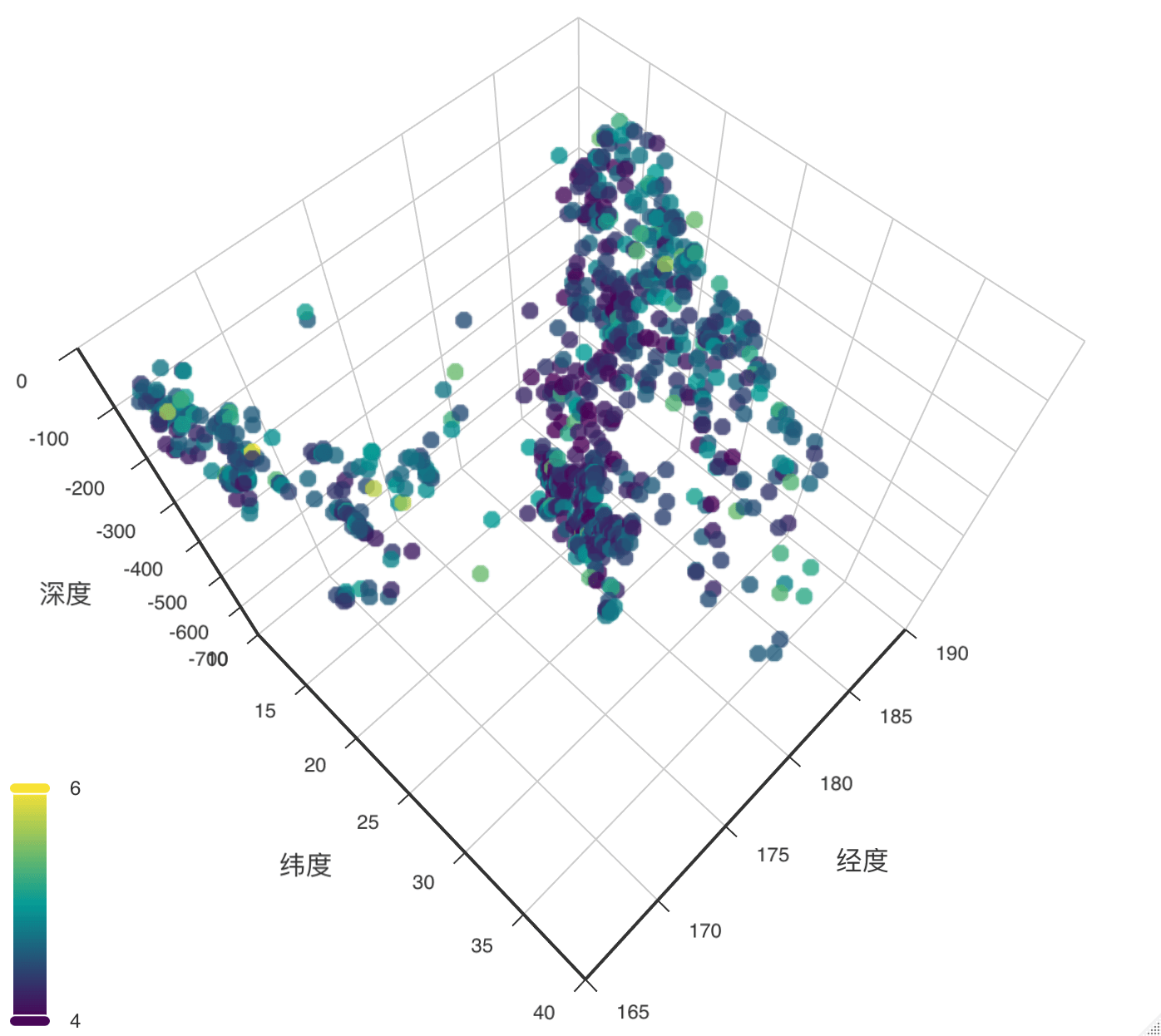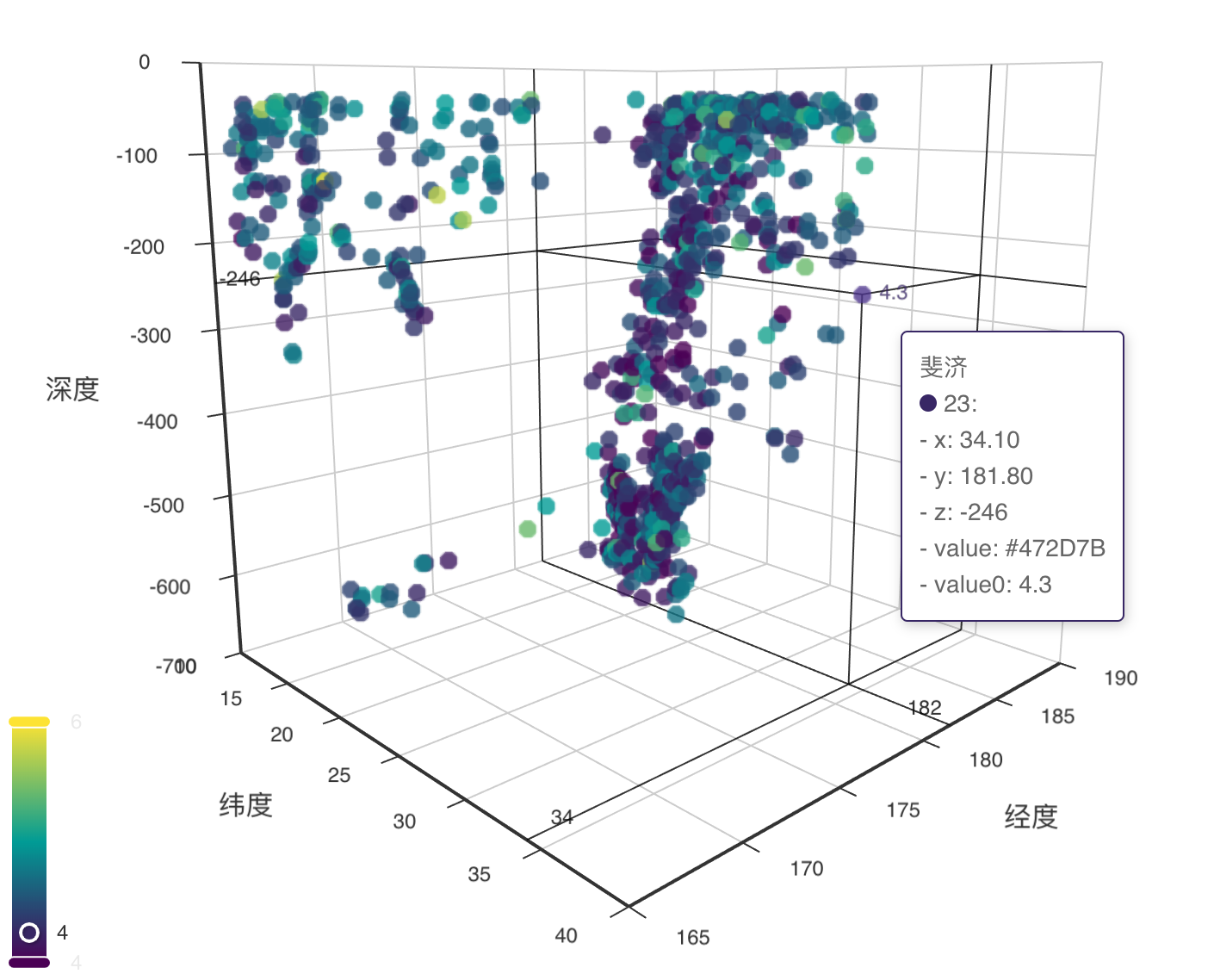# 1 概览

1. cranlogs 获取 ggplot2rglplotlylatticeecharts4r 包的每日的下载量，下图展示 2020-01-01 至 2022-12-31 的下载量趋势。

cran_downloads_pkgs <- readRDS(file = "data/cran_downloads_pkgs.rds")
library(ggplot2)
ggplot(data = cran_downloads_pkgs, aes(x = date, y = count, color = package)) +
geom_line() +
scale_color_discrete(breaks = c("ggplot2", "rgl", "plotly", "lattice", "echarts4r")) +
scale_y_continuous(n.breaks = 7,
labels = scales::label_number(scale_cut = scales::cut_short_scale())) +
scale_x_date(limits = as.Date(c("2020-01-01", "2023-01-01")), date_labels = "%B\n%Y") +
theme_classic()  +
coord_cartesian(expand = FALSE) +
labs(x = "日期", y = "下载次数", color = "R 包")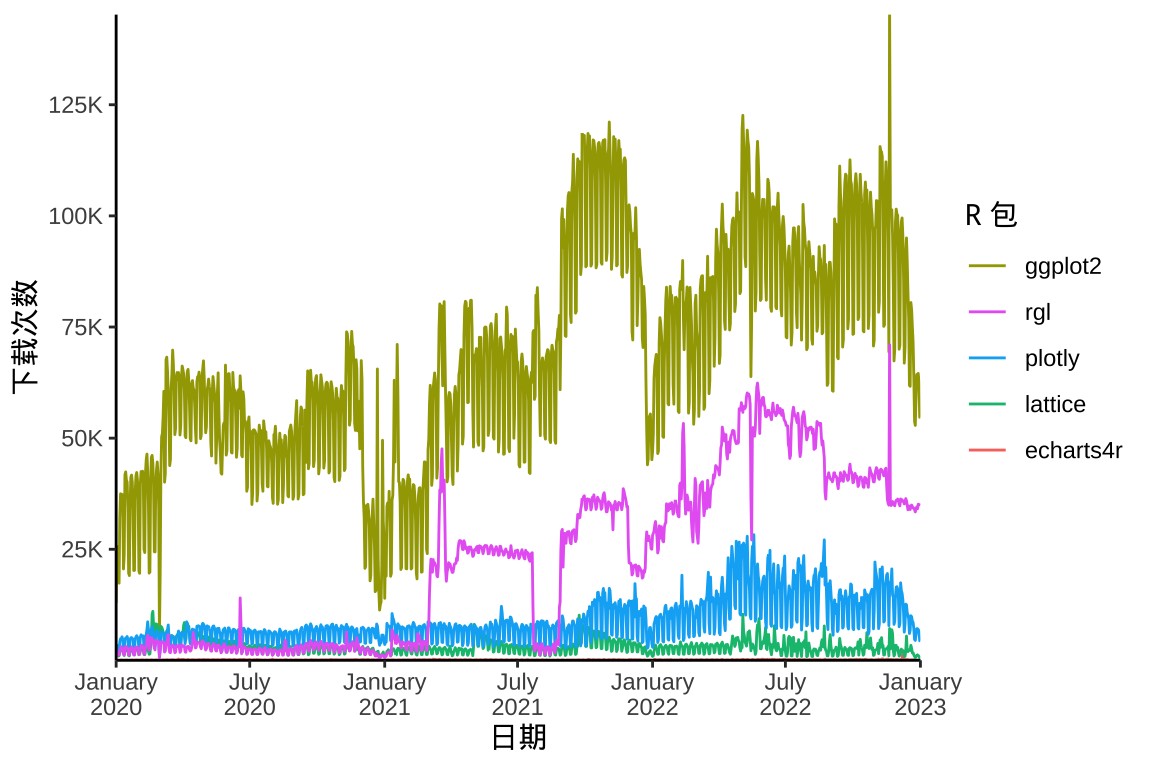图 1.1: 2020-2022 年下载量的趋势变化

2. Base R、latticeggplot2 三个作图工具已经提供了许多函数，支持的图形种类是不同的。下表中ggmosaic 包提供函数 geom_mosaic() 绘制马赛克图，ggally 包提供函数 ggpairs() 绘制矩阵图。latticetools 包提供函数 panel.piechart() 绘制饼图。

作用 图形 graphics lattice ggplot2
描述关系 散点图 plot xyplot geom_point
描述关系 三维散点图 scatterplot3d cloud -
描述关系 矩阵图 pairs splom ggpairs
描述关系 符号图 symbols panel.superpose geom_point
描述关系 二维平滑散点图 smoothScatter panel.smoothScatter geom_smooth
描述关系 向日葵图 sunflowerplot
描述关系 四瓣图 fourfoldplot
描述关系 关联图 assocplot
描述趋势 轮廓图 contour contourplot geom_contour
描述趋势 栅格图 image levelplot geom_raster
描述趋势 曲线图 curve panel.curve geom_curve
描述趋势 三维透视图 persp wireframe -
描述趋势 条件图 coplot xyplot geom_point
描述趋势 折线图 lines panel.lines geom_line
描述分布 直方图 hist histogram geom_histogram
描述分布 箱线图 boxplot bwplot geom_boxplot
描述分布 密度图 plot.density densityplot geom_density
描述分布 QQ 图 qqplot qq geom_qq
描述分布 一维散点图 stripchart stripplot geom_point
描述分布 茎叶图 stem
描述分布 条件密度图 cdplot densityplot geom_density
描述分布 轴须图 rug panel.rug geom_rug
描述对比 条形图 barplot barchart geom_bar
描述对比 克利夫兰点图 dotchart dotplot geom_point
描述对比 星图 stars
描述占比 饼图 pie panel.piechart geom_col
描述占比 堆积图 spineplot barchart geom_col
描述占比 马赛克图 mosaicplot geom_mosaic

Base R 的函数 plot() 是泛型函数，lattice 包的 xyplot() 也是。以绘制条件图为例，Base R 提供函数 coplot()lattice 包也支持公式语法，以竖线 | 分割主变量和条件变量，比如 ~ x | y，右侧符号 y 表示条件变量。

3. 三个作图工具的使用方法截然不同，提供 Base R 、lattice 和 ggplot2 的等价作图代码是一件不太容易的事，渲染出来的图片风格迥异。以条件密度图为例，Base R 的作图函数为 cdplot()lattice 包的作图函数为 densityplot()ggplot2 包的作图函数为 geom_density()，代码如下：

with(iris, cdplot(x = Sepal.Length, y = Species, bw = .2))
library(lattice)
densityplot(~ Sepal.Length, groups = Species,
data = iris, bw = .2, scales = "free")
library(ggplot2)
ggplot(iris, aes(x = Sepal.Length, y = after_stat(count))) +
geom_density(aes(fill = Species), position = "fill",
show.legend = FALSE, bw = .2)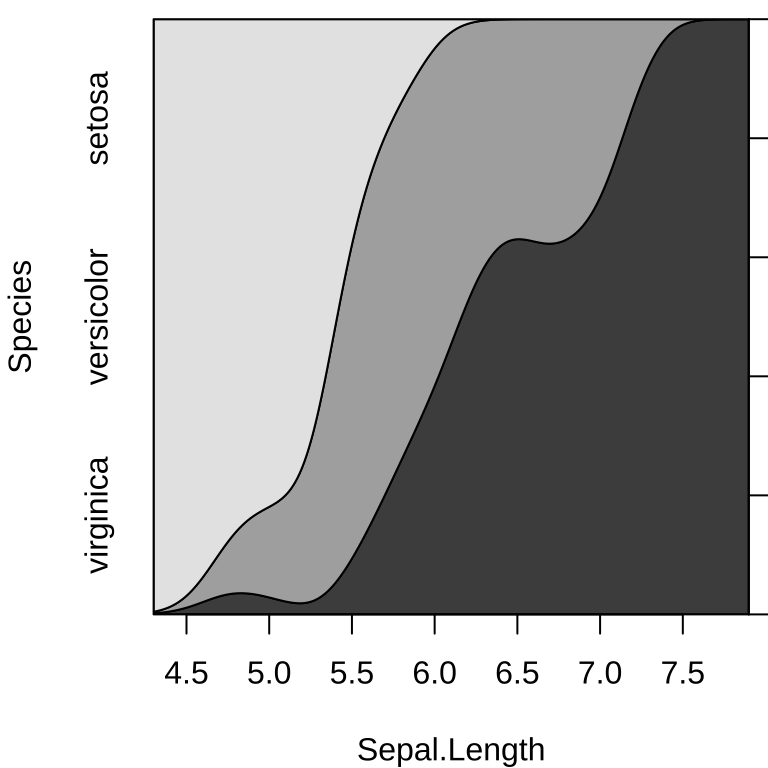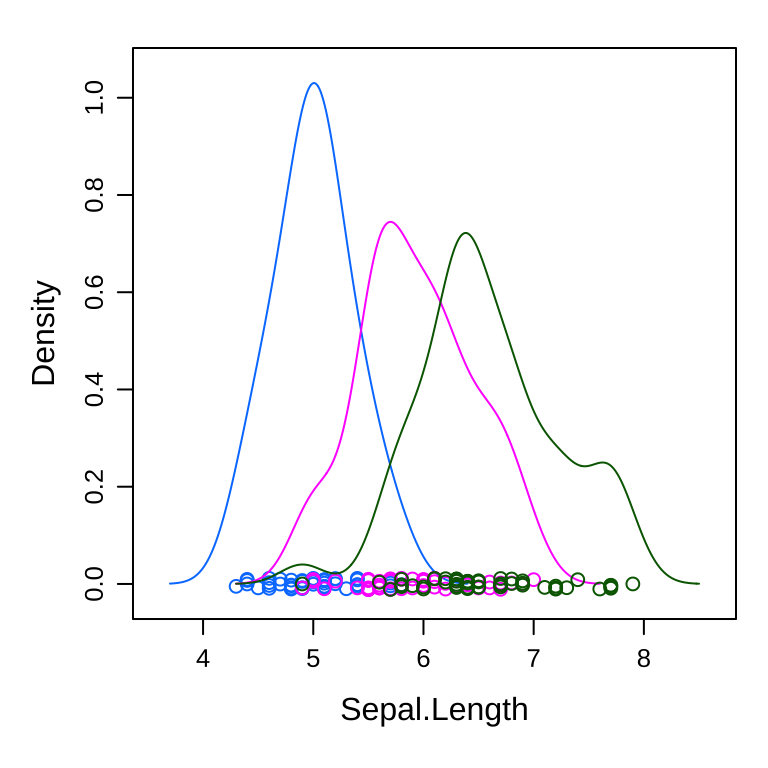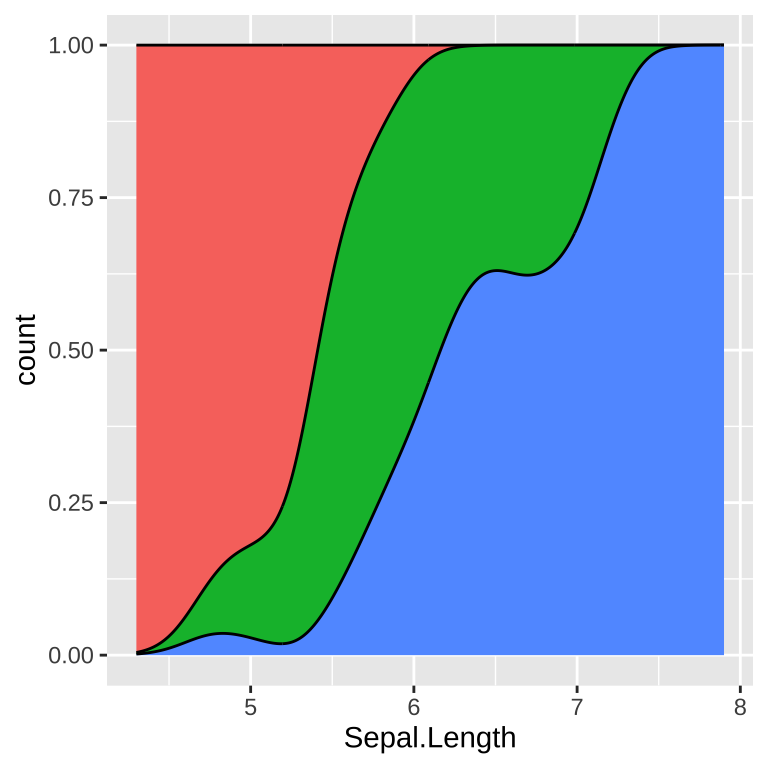图 1.2: 条件密度图

Base R 扩展包 scatterplot3d 可以绘制三维散点图，lattice 扩展包 latticeExtra 补充了很多绘图函数，见下表 。sp 包和 rasterVis 包 分别针对空间点和栅格数据，大大扩充了 lattice 在空间数据可视化方面的功能。

latticelatticeExtra 包的一些函数
R 包 函数 图形 作用
lattice parallelplot 平行坐标图 描述对比
lattice tmd Tukey 均值差异图 描述对比
latticeExtra ecdfplot 经验分布图 描述分布
latticeExtra rootogram Tukey 悬挂根图 描述分布
latticeExtra horizonplot 水平线图 描述趋势
latticeExtra mapplot 地区分布图 描述空间分布
latticeExtra tileplot Voronoi 图 描述空间分布
latticeExtra segplot 置信区间图 描述不确定性

# 2 非交互图形

## 2.1 Base R

# ggplot2 绘图
library(ggplot2)
ggplot(data = quakes, aes(x = long, y = lat)) + geom_point()
# Base R 绘图
plot(data = quakes, lat ~ long)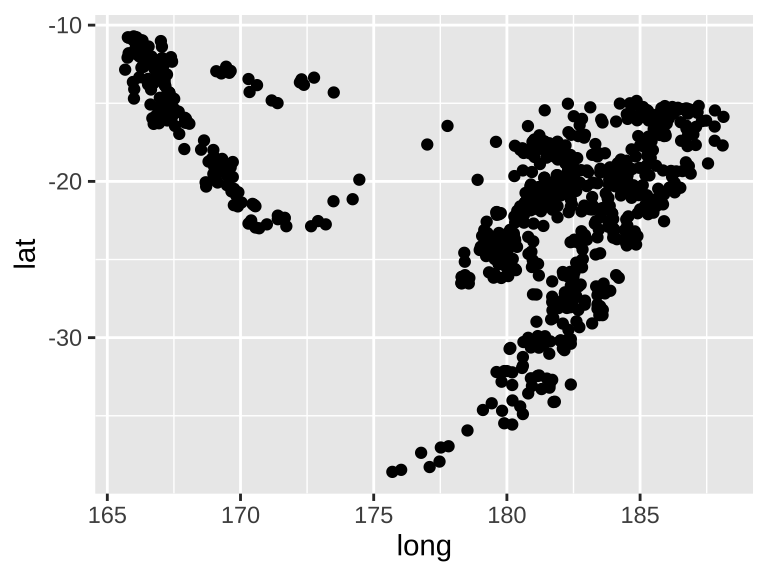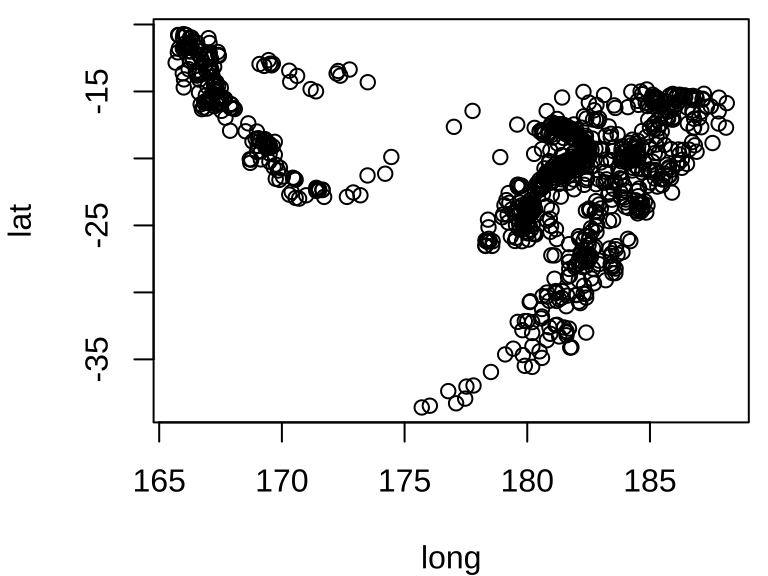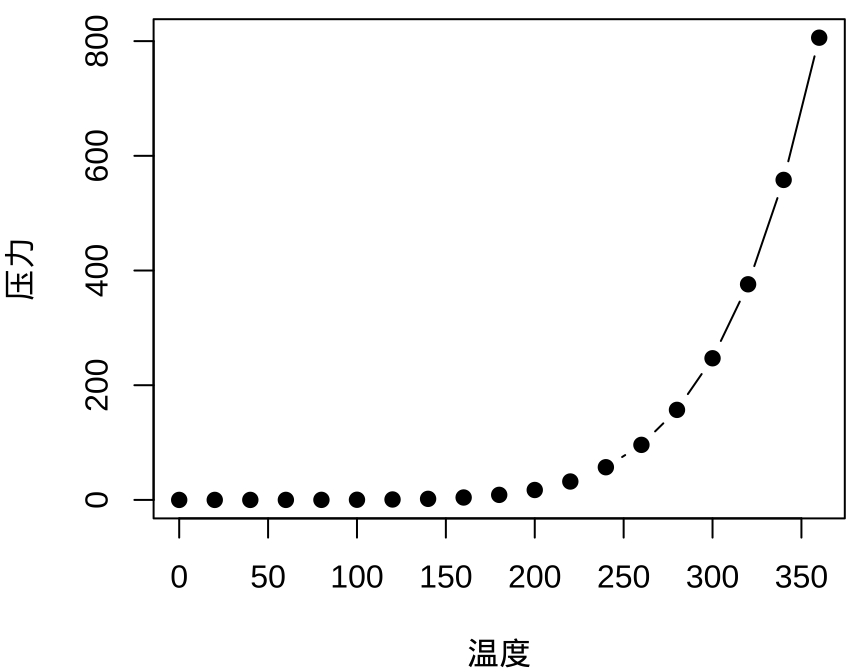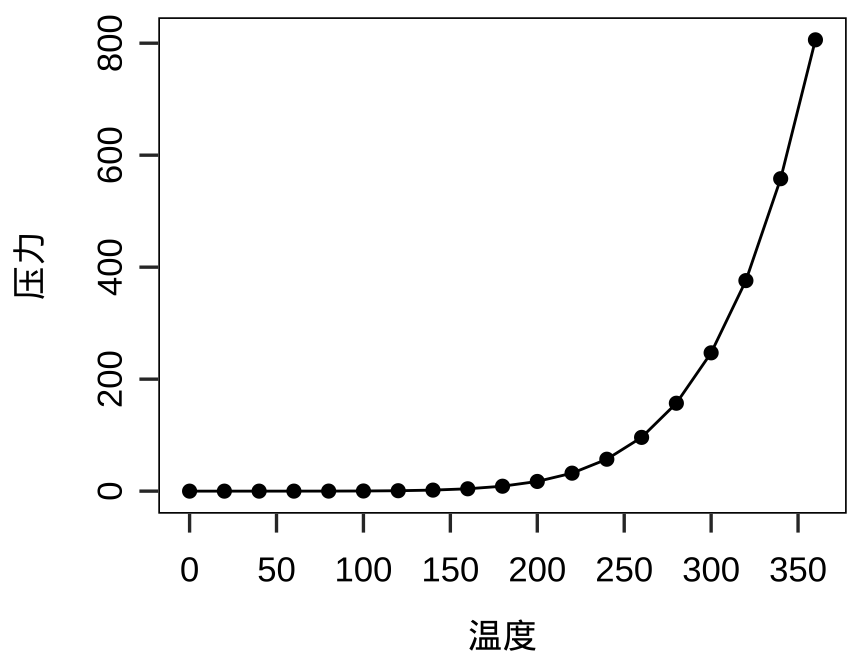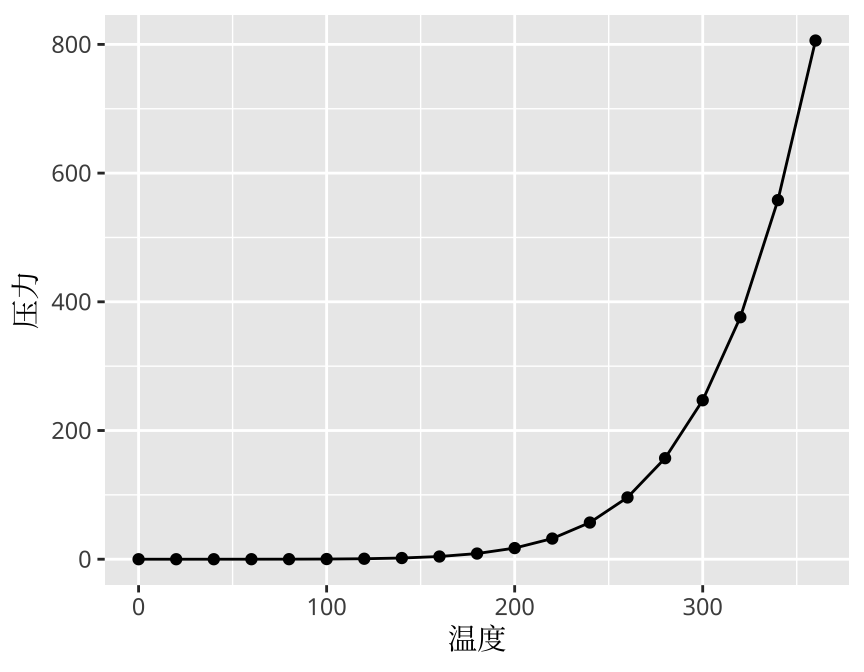-20.42 181.6 562 4.8 41
-20.62 181.0 650 4.2 15
-26.00 184.1 42 5.4 43
-17.97 181.7 626 4.1 19
-20.42 182.0 649 4.0 11
-19.68 184.3 195 4.0 12

scatterplot3d 包是基于 Base R 基础绘图系统的，专门用于三维可视化。

# 将连续型数据向量转化为颜色向量
colorize_numeric <- function(x) {
scales::col_numeric(palette = "viridis", domain = range(x))(x)
}
# 在数据集 quakes 上添加新的数据 color
quakes <- within(quakes, {
color <- colorize_numeric(mag)
})
# 三维散点图
library(scatterplot3d)
with(quakes, {
scatterplot3d(
x = long, y = lat, z = - depth,
color = color, pch = 20,
mar = c(3, 3, 0, 3) + 0.1,
xlab = "经度", ylab = "纬度", zlab = "深度"
)
})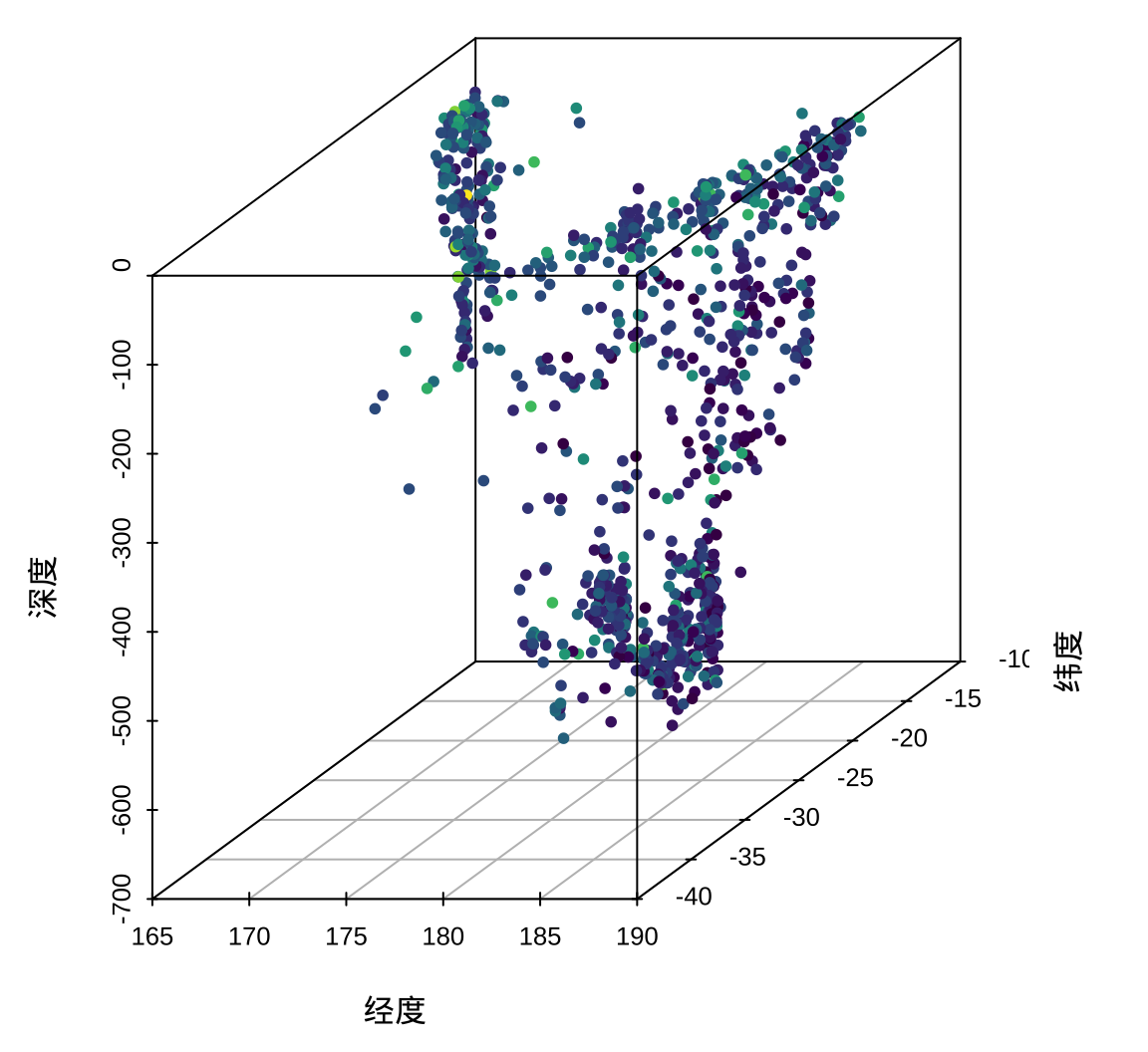## 2.2 lattice

lattice 包是继承自 grid 包的栅格绘图系统。 lattice 绘图的一般模式见《地区分布图及其应用》

library(lattice)
# 三维气泡图
cloud(-depth ~ long * lat,
data = quakes, pch = 19,
col = quakes$color, # 气泡颜色映射震级 cex = quakes$mag - 4, # 气泡大小映射震级
xlab = "经度",
ylab = "纬度",
zlab = list("深度", rot = 90),
# z 轴标签旋转 90 度
scales = list(
arrows = FALSE, col = "black",
z = list(rot = 90)
),
# 减少三维图形的边空
lattice.options = list(
layout.widths = list(
left.padding = list(x = -.6, units = "inches"),
right.padding = list(x = -1.0, units = "inches")
),
layout.heights = list(
bottom.padding = list(x = -.8, units = "inches"),
top.padding = list(x = -1.0, units = "inches")
)
),
# 设置坐标轴字体大小
par.settings = list(
axis.line = list(col = "transparent"),
fontsize = list(text = 15, points = 10)
),
# 设置三维图的观察方位
screen = list(z = 30, x = -65, y = 0)
)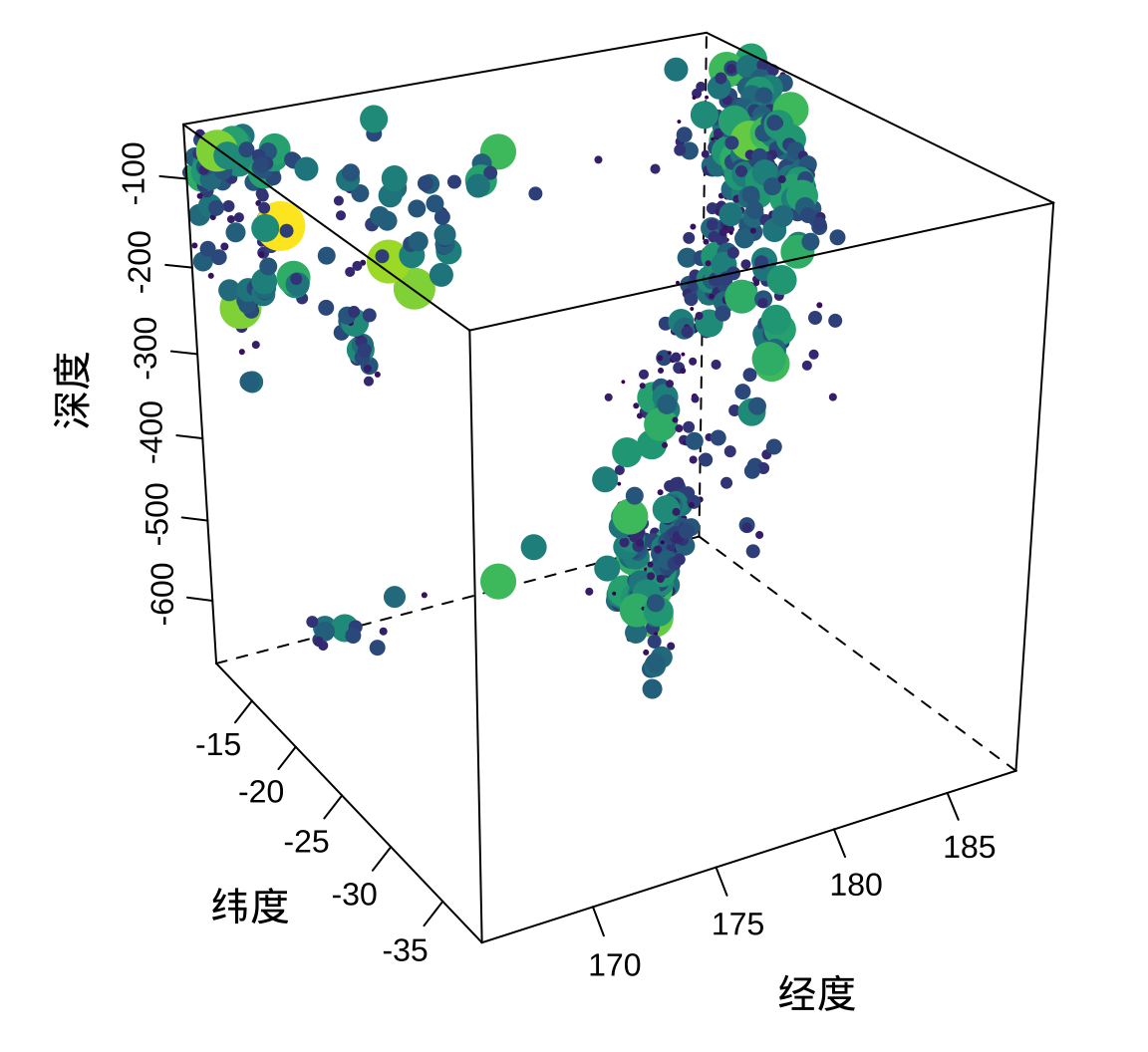• lattice.options 全局控制选项，比如绘图区域的边空布局等。
• par.settings 设置图形参数，比如轴线颜色，字体大小等。
• screen 设置三维图的观察方位，参数 xyz 分别设置数据绕 x 轴、y 轴和 z 轴旋转的角度，负数表示按逆时针旋转。
• scales 设置坐标轴上的刻度，如刻度线的颜色，刻度标签位置。

## 2.3 ggplot2

ggplot2 是一个 R 语言扩展包，专用于绘制各种各样的统计图形，是数据探索和可视化的利器。2007 年 6 月 1 日 ggplot2 在 CRAN 上发布第一个正式版本 0.5，截止写作时间，ggplot2 已经持续迭代 10 多年了，发布了 40 多个版本，形成了一个非常庞大的生态，直接依赖 ggplot2 的 R 包接近 3000 个。从如下三个地方，可以窥见 ggplot2 生态的一角，感受其魅力。

• Daniel Emaasit 收集了 110 多个 ggplot2 衍生包，维护了一个 网站，统一组织、展示这些 R 包。本文会精心挑选一些高质量的 R 包予以介绍。

• Tom Mock 发起的 tidytuesday 项目吸引了数以千计的数据科学爱好者参与数据分析、探索和可视化项目，涌现了一批批优秀的基于 ggplot2 的可视化作品，极大地提升了 ggplot2 生态的影响力。本文也会基于真实的数据介绍每一个统计图形。

• Yan Holtz 整理了数以百计的统计图形，分门别类地在网站上展示，方便读者预览效果、选择合适的图形。也是受该网站启发，本文在介绍完 ggplot2 绘图的基础要素后，从统计图形的作用出发，按照趋势、关系、占比、对比、分布和不确定性等六大方面予以介绍。

# 3 交互式图形

R 语言社区有很多 R 包可以绘制动态交互图形，有的 R 包功能非常综合，如 echarts4r 包、 plotly 包等，有的 R 包功能非常专门化，如 rgl 包和 leaflet包。

## 3.1 OpenGL 与 rgl

rgl 是制作三维交互图形的专门化 R 包。相比于用函数 persp() 制作的三维透视图，rgl 算是非常的实现三维图形绘制的 R 包，它在 2004 年就发布在 CRAN 上了，时间一晃，18 年过去了。

mikefc 戏称自己造了中看不中用的 ggrgl 包，其 Github ID 正是 coolbutuseless，按字面意思拆开来就是「cool but useless」，不过，mikefc 的个人博客干货很多，而且非常高产，推荐读者看看。这让我一下想到贾宝玉在假山下和林黛玉一起偷看《西厢记》的场景，宝玉问：银样蜡枪头是什么意思？黛玉回答说：中看不中用。有些图形实在没有必要升维做成立体的，比如条形图或柱形图，一些反面例子可以在漫谈条形图你问我答两篇文章中找到。

rayrender 不依赖 rgl 包，主打 3D 建模，渲染复杂场景，没有外部依赖，可制作三维立体感的 ggplot2 图形，推荐其替代 rgl 制作三维动画。rayshader 依赖 rglrayrender 等提供阴影特效，适合地形景观图，Tyler Morgan-Wall 也曾在 RStudio 大会上介绍过 rayshader，Github 星赞超过 1500，算是比较流行的 R 包了。

imagemagick 是一个独立的图像后期处理软件，它可以将一系列静态图片合成 GIF 动图，magick 是 Jeroen Ooms 开发的又一 R 接口包，ffmpeg 是一个独立的视频处理软件。

LaTeX 宏包 animate，常用于 beamer 幻灯片或 PDF 文档中，将一系列 PNG/PDF 图片合成动画，就是将一幅幅图片以快速地方式播放，形成动画效果，需要使用 Adobe 阅读器播放才可见效果。

# 将连续型数据向量转化为颜色向量
colorize_numeric <- function(x) {
scales::col_numeric(palette = "viridis", domain = range(x))(x)
}
library(rgl)
# 设置视角
view3d(
theta = 30, phi = 45, fov = 30,
zoom = 1, interactive = TRUE
)
# 绘制图形
with(quakes, {
# 在数据集 quakes 上添加新的数据 color
color <- colorize_numeric(mag)
plot3d(
x = long, y = lat, z = -depth,
xlab = "经度",
ylab = "纬度",
zlab = "深度",
col = color, size = mag - 4, type = "s"
)
})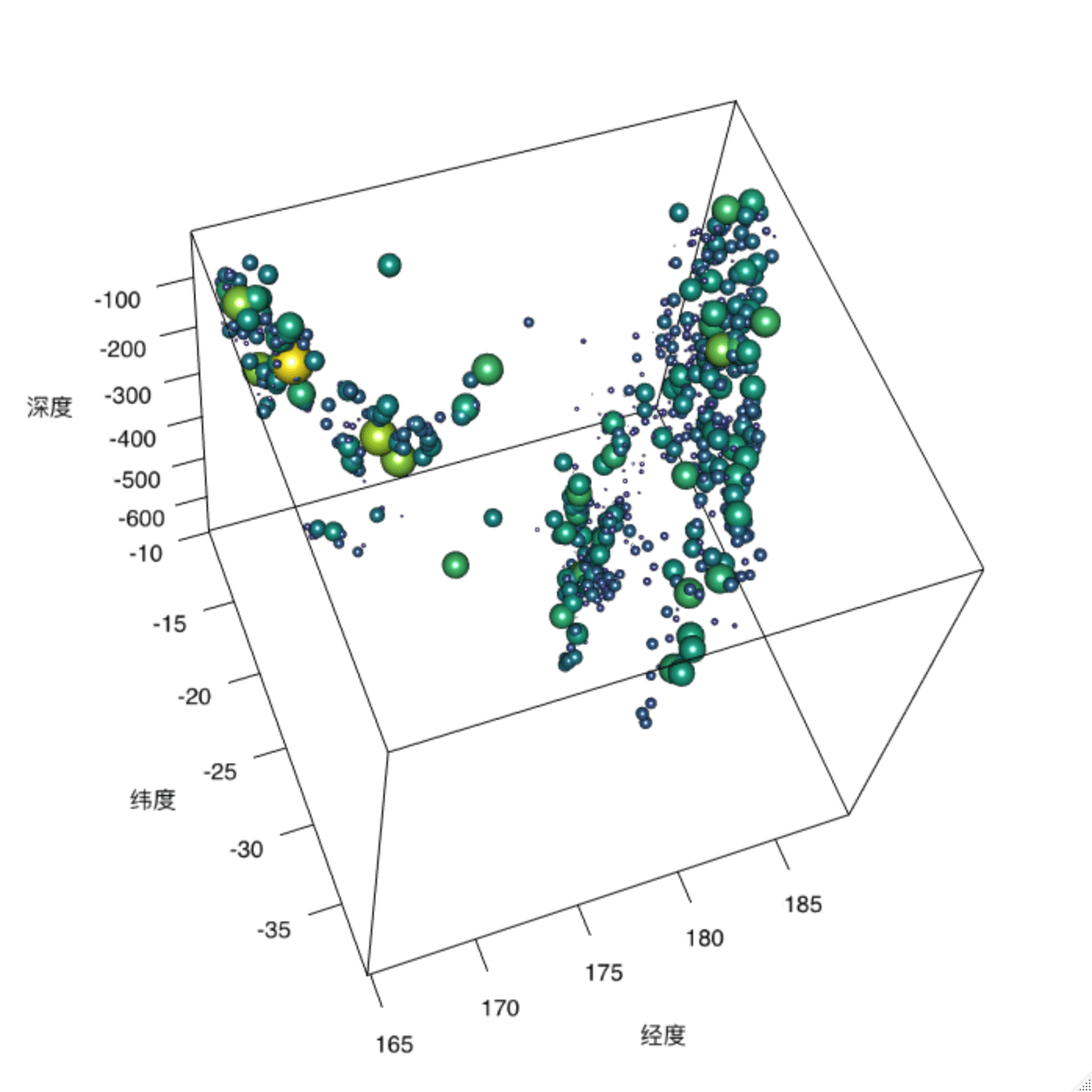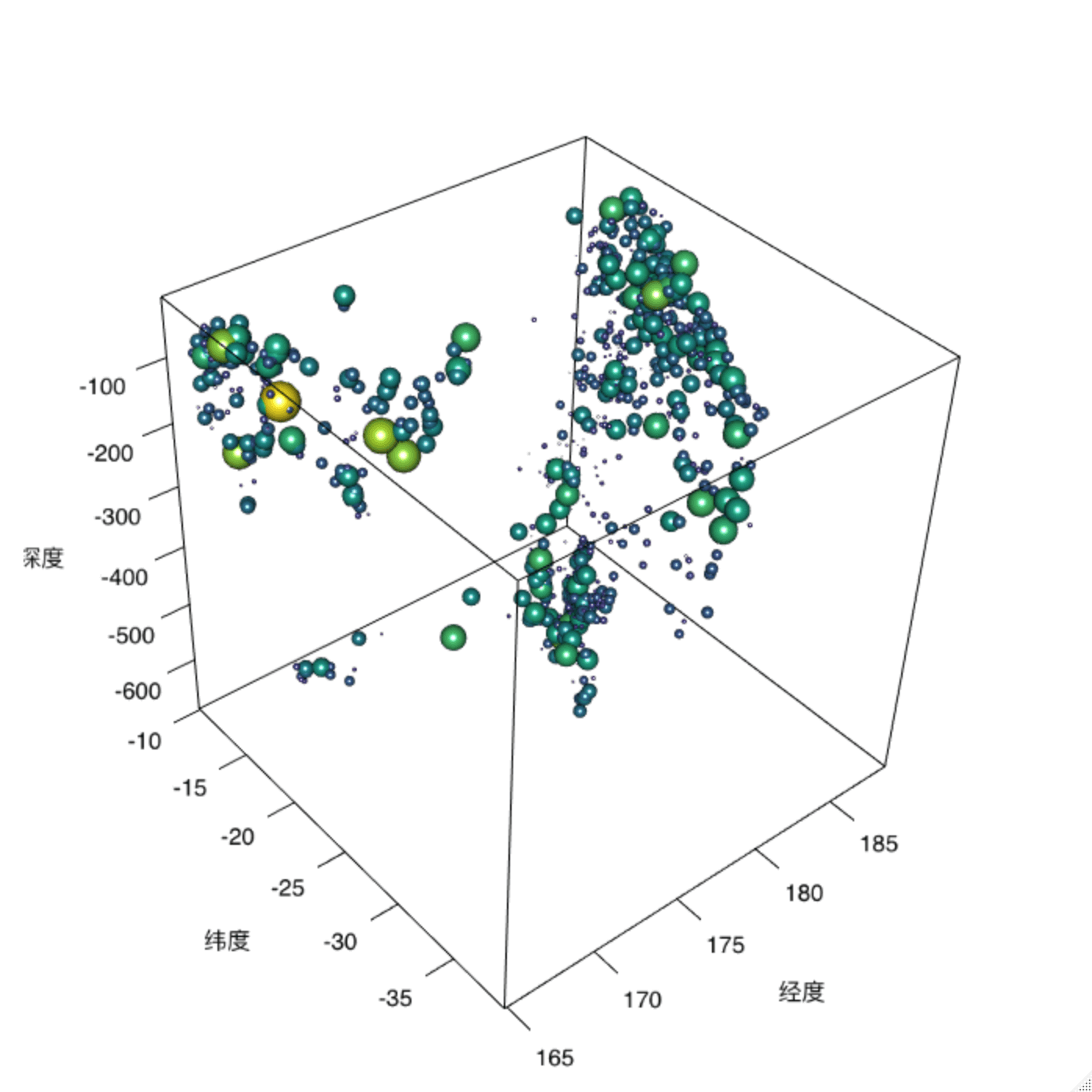## 3.2 WebGL 与 plotly

WebGL是一种 JavaScript API，可以使用计算机的 GPU 硬件资源加速 2D 和 3D 图形渲染，2011 年发布 1.0 规范，2017 年完成 2.0 规范，目前，主流浏览器 Safari、Chrome、Edge 和 Firefox 等均已支持。Google 搜索在 2012 年应用了这一技术，只要在搜索框内输入一个函数曲线，比如 arcsin(x*y)/(x*y)，那么结果页会展示这个图像。

plotly.js 提供很多图层用于绘制各类图形，见plotly.js 源码库，其中支持 WebGL 的有热力图 heatmapgl、 散点图 scattergl 和极坐标系下的散点图 scatterpolargl

plotly 可以与 ggplot2 紧密结合，提供函数 ggplotly() 直接将静态的 ggplot2 图形转化为交互式的 plotly 图形，函数 plot_ly() 也提供一致的使用语法。

# 更多详情见 https://plot.ly/r/reference/#scatter3d
plotly::plot_ly(
data = quakes, x = ~long, y = ~lat, z = ~depth,
type = "scatter3d", mode = "markers",
marker = list(
size = ~mag, color = ~mag,
colorscale = "Viridis",
colorbar = list(title = list(text = "震级"))
)
) |>
plotly::layout(scene = list(
xaxis = list(title = "经度"),
yaxis = list(title = "纬度"),
zaxis = list(title = "深度")
))

plotly 团队开发了 plotly.js 库，且维护了 R 接口文档 (https://plotly.com/r/)，Carson Sievert 开发了 plotly 包，配套书 Interactive web-based data visualization with R, plotly, and shiny。 Paul C. Bauer 的书 Applied Data Visualization 介绍 plotly https://bookdown.org/paul/applied-data-visualization/what-is-plotly.html

plotly 包的函数使用起来还是比较复杂的，特别是需要打磨细节以打造数据产品时，此外，其依赖相当重，仅数据处理就包含两套方法 — dplyr 和 data.table，引起很多函数冲突，可谓「苦其久矣」！因此，准备另起炉灶，开发一个新的 R 包 qplotly，取意 quick plotly，以 qplot_ly() 替代 plot_ly()。类似简化 API 的工作有 simplevisautoplotlyggfortifyplotme

## 3.3 echarts4r 等

plotly 包类似，echarts4r 是另一个流行的 JavaScript 绘图库的 R 语言接口，同样支持非常广泛的图形种类。echarts4r 是 Apache Echarts 的 R 语言接口。Apache Echarts 支持 WebGL 的图形有散点图、路径图、矢量场图、网络图，详见官方示例文档，大规模的数据可视化需要比较好的硬件资源支持。

ggiraph 可以与 ggplot2 包 紧密结合，提供一致的使用语法，结合 usmap 包可以制作地图，悬浮提示可以包含文字、链接，与 gt 包结合，悬浮提示还可以包含图片、表格等元素。

mapdeck 提供了 Deck.glMapbox 的 R 语言接口，Deck.gl 号称是 WebGL 2.0 加持的高性能大规模数据可视化渲染组件，也是以 MIT 协议开源的软件，而后者是商业收费软件。rdeck 类似 mapdeck，但是提供更多实用的功能，优化的数据存储方式，过滤了不可见的数据，更加友好的配色主题，适用于图例和悬浮提示等。 Kyle Walker 开发了 mapboxapi 包，也提供 Mapbox Web 服务的 R 语言接口。

reticulate 将 Python 社区的绘图模块引入 R 语言社区，比较流行的交互式绘图模块有 pyechartsplotly.pybokeh 等。

library(echarts4r)
# 待 echarts4r 发布 0.4.5 版后，升级 echarts4r
# 不再需要添加 color 列为 mag
quakes$color <- quakes$mag
# 绘制图形
quakes |>
e_charts(x = lat) |>
e_scatter_3d(
y = long, z = depth,
size = mag,
color = mag,
bind = stations,
coordinate_system = "cartesian3D",
name = "斐济"
) |>
e_x_axis_3d(min = -40, max = -10, name = "纬度") |>
e_y_axis_3d(min = 165, max = 190, name = "经度") |>
e_z_axis_3d(name = "深度") |>
e_visual_map(
serie = mag,
type = "continuous",
inRange = list(color = c('#4B0055', '#009B95', '#FDE333')),
dimension = 4, # third dimension x = 0, y = 1, z = 2, color = 3, size = 4
top = 20
) |>
e_visual_map(
serie = mag,
type = "continuous",
inRange = list(symbolSize = c(5, 15)),
dimension = 3,
bottom = 10
) |>
e_tooltip() |>
e_title(text = "斐济及其周边地震活动")